# class TSpectrum2: public TNamed

```THIS CLASS CONTAINS ADVANCED SPECTRA PROCESSING FUNCTIONS.

ONE-DIMENSIONAL BACKGROUND ESTIMATION FUNCTIONS
TWO-DIMENSIONAL BACKGROUND ESTIMATION FUNCTIONS
ONE-DIMENSIONAL SMOOTHING FUNCTIONS
TWO-DIMENSIONAL SMOOTHING FUNCTIONS
ONE-DIMENSIONAL DECONVOLUTION FUNCTIONS
TWO-DIMENSIONAL DECONVOLUTION FUNCTIONS
ONE-DIMENSIONAL PEAK SEARCH FUNCTIONS
TWO-DIMENSIONAL PEAK SEARCH FUNCTIONS

These functions were written by:
Miroslav Morhac
Institute of Physics
Dubravska cesta 9, 842 28 BRATISLAVA
SLOVAKIA

email:fyzimiro@savba.sk,    fax:+421 7 54772479

The original code in C has been repackaged as a C++ class by R.Brun

The algorithms in this class have been published in the following
references:
  M.Morhac et al.: Background elimination methods for
multidimensional coincidence gamma-ray spectra. Nuclear
Instruments and Methods in Physics Research A 401 (1997) 113-
132.

  M.Morhac et al.: Efficient one- and two-dimensional Gold
deconvolution and its application to gamma-ray spectra
decomposition. Nuclear Instruments and Methods in Physics
Research A 401 (1997) 385-408.

  M.Morhac et al.: Identification of peaks in multidimensional
coincidence gamma-ray spectra. Nuclear Instruments and Methods in
Research Physics A  443(2000), 108-125.

These NIM papers are also available as Postscript files from:

ftp://root.cern.ch/root/SpectrumDec.ps.gz
ftp://root.cern.ch/root/SpectrumSrc.ps.gz
ftp://root.cern.ch/root/SpectrumBck.ps.gz

NEW FUNCTIONS  January 2006

```

All figures in this page were prepared using DaqProVis system, Data Acquisition, Processing and Visualization system, which is being developed at the Institute of Physics, Slovak Academy of Sciences, Bratislava, Slovakia:

http://www.fu.sav.sk/nph/projects/DaqProVis/ under construction

## Function Members (Methods)

public:
protected:
 virtual void TObject::DoError(int level, const char* location, const char* fmt, va_list va) const void TObject::MakeZombie()

## Data Members

public:
 enum { kBackIncreasingWindow kBackDecreasingWindow kBackSuccessiveFiltering kBackOneStepFiltering }; enum TObject::EStatusBits { kCanDelete kMustCleanup kObjInCanvas kIsReferenced kHasUUID kCannotPick kNoContextMenu kInvalidObject }; enum TObject::[unnamed] { kIsOnHeap kNotDeleted kZombie kBitMask kSingleKey kOverwrite kWriteDelete };
protected:
 TH1* fHistogram resulting histogram Int_t fMaxPeaks Maximum number of peaks to be found Int_t fNPeaks number of peaks found TString TNamed::fName object identifier Float_t* fPosition [fNPeaks] array of current peak positions Float_t* fPositionX [fNPeaks] X position of peaks Float_t* fPositionY [fNPeaks] Y position of peaks Float_t fResolution resolution of the neighboring peaks TString TNamed::fTitle object title static Int_t fgAverageWindow Average window of searched peaks static Int_t fgIterations Maximum number of decon iterations (default=3)

## Class Charts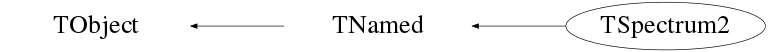## Function documentation

``` Constructor.
```
TSpectrum2(Int_t maxpositions, Float_t resolution = 1)
```  maxpositions:  maximum number of peaks
resolution:    determines resolution of the neighboring peaks
default value is 1 correspond to 3 sigma distance
between peaks. Higher values allow higher resolution
(smaller distance between peaks.
May be set later through SetResolution.
```

``` Destructor.
```
void SetAverageWindow(Int_t w = 3)
``` static function: Set average window of searched peaks
see TSpectrum2::SearchHighRes
```
void SetDeconIterations(Int_t n = 3)
``` static function: Set max number of decon iterations in deconvolution operation
see TSpectrum2::SearchHighRes
```
TH1 * Background(const TH1* hist, int niter = 20, Option_t* option = "")
```TWO-DIMENSIONAL BACKGROUND ESTIMATION FUNCTION
This function calculates the background spectrum in the input histogram h.
The background is returned as a histogram.

Function parameters:
-h: input 2-d histogram
-numberIterations, (default value = 20)
Increasing numberIterations make the result smoother and lower.
-option: may contain one of the following options
- to set the direction parameter
"BackIncreasingWindow". By default the direction is BackDecreasingWindow
- filterOrder-order of clipping filter,  (default "BackOrder2"
-possible values= "BackOrder4"
"BackOrder6"
"BackOrder8"
- "nosmoothing"- if selected, the background is not smoothed
By default the background is smoothed.
- smoothWindow-width of smoothing window, (default is "BackSmoothing3")
-possible values= "BackSmoothing5"
"BackSmoothing7"
"BackSmoothing9"
"BackSmoothing11"
"BackSmoothing13"
"BackSmoothing15"
- "Compton" if selected the estimation of Compton edge
will be included.
- "same" : if this option is specified, the resulting background
histogram is superimposed on the picture in the current pad.

NOTE that the background is only evaluated in the current range of h.
ie, if h has a bin range (set via h->GetXaxis()->SetRange(binmin,binmax),
the returned histogram will be created with the same number of bins
as the input histogram h, but only bins from binmin to binmax will be filled
with the estimated background.

```
void Print(Option_t* option = "") const
``` Print the array of positions
```
Int_t Search(const TH1* hist, Double_t sigma = 2, Option_t* option = "", Double_t threshold = 0.05)
```TWO-DIMENSIONAL PEAK SEARCH FUNCTION
This function searches for peaks in source spectrum in hin
The number of found peaks and their positions are written into
the members fNpeaks and fPositionX.
The search is performed in the current histogram range.

Function parameters:
hin:       pointer to the histogram of source spectrum
sigma:   sigma of searched peaks, for details we refer to manual
threshold: (default=0.05)  peaks with amplitude less than

By default, the background is removed before deconvolution.
Specify the option "nobackground" to not remove the background.       //

By default the "Markov" chain algorithm is used.
Specify the option "noMarkov" to disable this algorithm
Note that by default the source spectrum is replaced by a new spectrum//

By default a polymarker object is created and added to the list of
functions of the histogram. The histogram is drawn with the specified
option and the polymarker object drawn on top of the histogram.
The polymarker coordinates correspond to the npeaks peaks found in
the histogram.
A pointer to the polymarker object can be retrieved later via:
TList *functions = hin->GetListOfFunctions();
TPolyMarker *pm = (TPolyMarker*)functions->FindObject("TPolyMarker")
Specify the option "goff" to disable the storage and drawing of the
polymarker.

```
void SetResolution(Float_t resolution = 1)
```  resolution: determines resolution of the neighboring peaks
default value is 1 correspond to 3 sigma distance
between peaks. Higher values allow higher resolution
(smaller distance between peaks.
May be set later through SetResolution.
```
const char * Background(float** spectrum, Int_t ssizex, Int_t ssizey, Int_t numberIterationsX, Int_t numberIterationsY, Int_t direction, Int_t filterType)
```TWO-DIMENSIONAL BACKGROUND ESTIMATION FUNCTION - RECTANGULAR RIDGES
This function calculates background spectrum from source spectrum.
The result is placed to the array pointed by spectrum pointer.

Function parameters:
spectrum-pointer to the array of source spectrum
ssizex-x length of spectrum
ssizey-y length of spectrum
numberIterationsX-maximal x width of clipping window
numberIterationsY-maximal y width of clipping window
for details we refer to manual
direction- direction of change of clipping window
- possible values=kBackIncreasingWindow
kBackDecreasingWindow
filterType-determines the algorithm of the filtering
-possible values=kBackSuccessiveFiltering
kBackOneStepFiltering

```

Background estimation

Goal: Separation of useful information (peaks) from useless information (background)

         method is based on Sensitive Nonlinear Iterative Peak (SNIP) clipping algorithm 

         there exist two algorithms for the estimation of new value in the channel 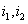

Algorithm based on Successive Comparisons

It is an extension of one-dimensional SNIP algorithm to another dimension. For details we refer to .

Algorithm based on One Step Filtering

New value in the estimated channel is calculated as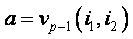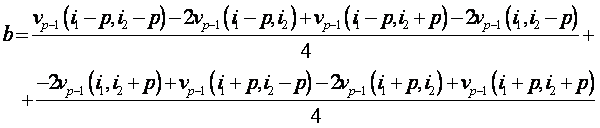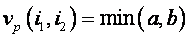.

where p = 1, 2, , number_of_iterations.

Function:

const char* TSpectrum2::Background (float **spectrum, int ssizex, int ssizey, int numberIterationsX, int numberIterationsY, int direction, int filterType)

This function calculates background spectrum from the source spectrum.  The result is placed in the matrix pointed by spectrum pointer.  One can also switch the direction of the change of the clipping window and to select one of the two above given algorithms. On successful completion it returns 0. On error it returns pointer to the string describing error.

Parameters:

spectrum-pointer to the matrix of source spectrum

ssizex, ssizey-lengths of the spectrum matrix

numberIterationsX, numberIterationsYmaximal widths of clipping

window,

direction- direction of change of clipping window

- possible values=kBackIncreasingWindow

kBackDecreasingWindow

filterType-type of the clipping algorithm,

-possible values=kBack SuccessiveFiltering

kBackOneStepFiltering

References:

  C. G Ryan et al.: SNIP, a statistics-sensitive background treatment for the quantitative analysis of PIXE spectra in geoscience applications. NIM, B34 (1988), 396-402.

  M. Morháč, J. Kliman, V. Matouek, M. Veselský, I. Turzo.: Background elimination methods for multidimensional gamma-ray spectra. NIM, A401 (1997) 113-132.

```
```

Example 1 script Back_gamma64.c :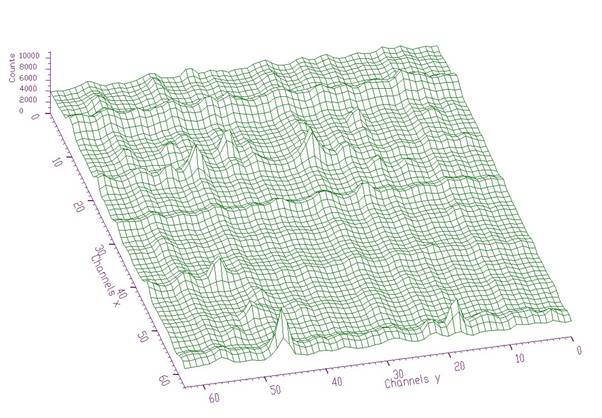Fig. 1 Original two-dimensional gamma-gamma-ray spectrum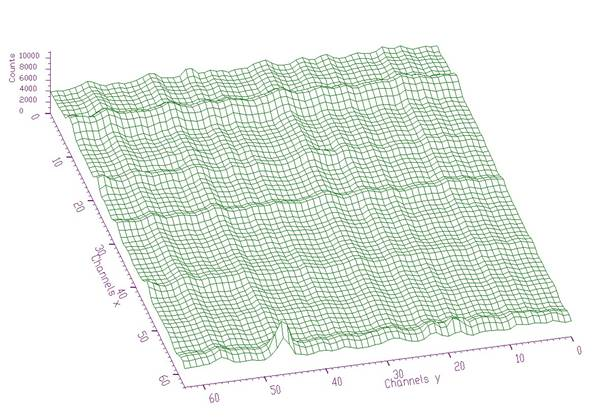Fig. 2 Background estimated from data from Fig. 1 using decreasing clipping window with widths 4, 4 and algorithm based on successive comparisons. The estimate includes not only continuously changing background but also one-dimensional ridges.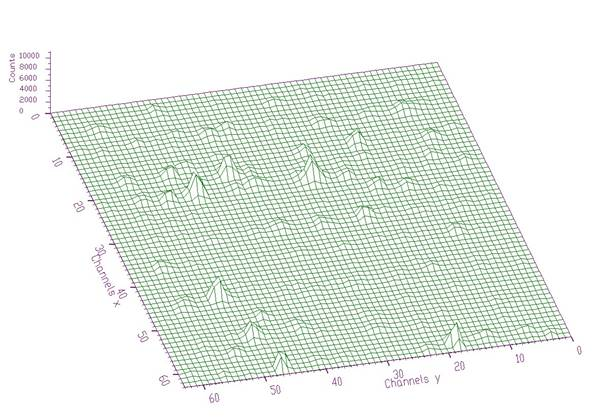Fig. 3 Resulting peaks after subtraction of the estimated background (Fig. 2) from original two-dimensional gamma-gamma-ray spectrum (Fig. 1).

Script:

// Example to illustrate the background estimator (class TSpectrum).

// To execute this example, do

// root > .x Back_gamma64.C

#include <TSpectrum>

void Back_gamma64() {

Int_t i, j;

Double_t nbinsx = 64;

Double_t nbinsy = 64;

Double_t xmin  = 0;

Double_t xmax  = (Double_t)nbinsx;

Double_t ymin  = 0;

Double_t ymax  = (Double_t)nbinsy;

Float_t ** source = new float *[nbinsx];

for (i=0;i<nbinsx;i++)

source[i]=new float[nbinsy];

TH2F *back = new TH2F("back","Background estimation",nbinsx,xmin,xmax,nbinsy,ymin,ymax);

TFile *f = new TFile("spectra2\\TSpectrum2.root");

back=(TH2F*) f->Get("back1;1");

TCanvas *Background = new TCanvas("Background","Estimation of background with increasing window",10,10,1000,700);

TSpectrum *s = new TSpectrum();

for (i = 0; i < nbinsx; i++){

for (j = 0; j < nbinsy; j++){

source[i][j] = back->GetBinContent(i + 1,j + 1);

}

}

s->Background(source,nbinsx,nbinsy,4,4,kBackDecreasingWindow,kBackSuccessiveFiltering);

for (i = 0; i < nbinsx; i++){

for (j = 0; j < nbinsy; j++)

back->SetBinContent(i + 1,j + 1, source[i][j]);

}

back->Draw("SURF");

}

Example 2 script Back_gamma256.c :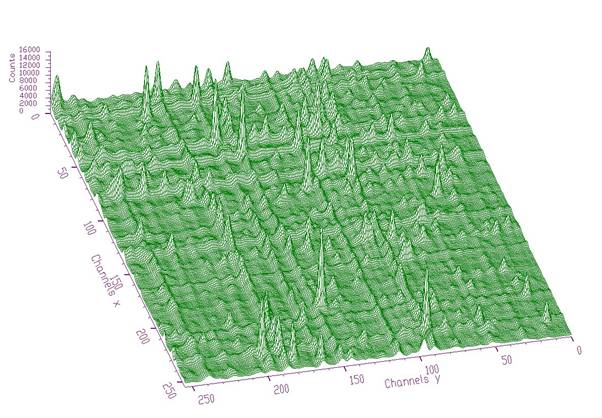Fig. 4 Original two-dimensional gamma-gamma-ray spectrum 256x256 channelsFig. 5 Peaks after subtraction of the estimated background (increasing clipping window with widths 8, 8 and algorithm based on successive comparisons) from original two-dimensional gamma-gamma-ray spectrum (Fig. 4).

Script:

// Example to illustrate the background estimator (class TSpectrum).

// To execute this example, do

// root > .x Back_gamma256.C

#include <TSpectrum>

void Back_gamma256() {

Int_t i, j;

Double_t nbinsx = 64;

Double_t nbinsy = 64;

Double_t xmin  = 0;

Double_t xmax  = (Double_t)nbinsx;

Double_t ymin  = 0;

Double_t ymax  = (Double_t)nbinsy;

Float_t ** source = new float *[nbinsx];

for (i=0;i<nbinsx;i++)

source[i]=new float[nbinsy];

TH2F *back = new TH2F("back","Background estimation",nbinsx,xmin,xmax,nbinsy,ymin,ymax);

TFile *f = new TFile("spectra2\\TSpectrum2.root");

back=(TH2F*) f->Get("back2;1");

TCanvas *Background = new TCanvas("Background","Estimation of background with increasing window",10,10,1000,700);

TSpectrum *s = new TSpectrum();

for (i = 0; i < nbinsx; i++){

for (j = 0; j < nbinsy; j++){

source[i][j] = back->GetBinContent(i + 1,j + 1);

}

}

s->Background(source,nbinsx,nbinsy,8,8,kBackIncreasingWindow,kBackSuccessiveFiltering);

for (i = 0; i < nbinsx; i++){

for (j = 0; j < nbinsy; j++)

back->SetBinContent(i + 1,j + 1, source[i][j]);

}

back->Draw("SURF");

}

Example 3 script Back_synt256.c :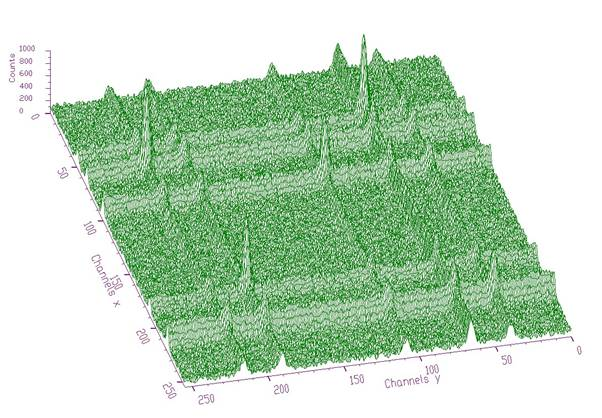Fig. 6 Original two-dimensional synthetic spectrum 256x256 channels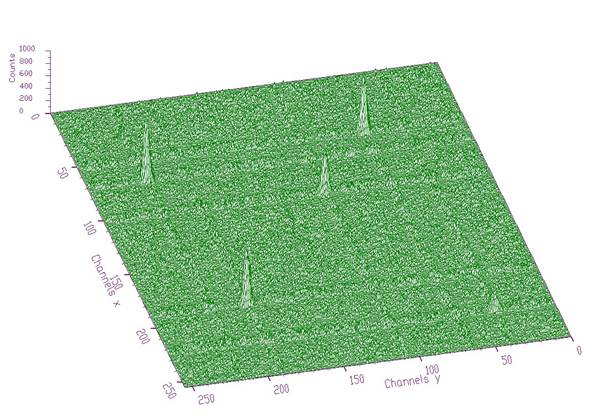Fig. 7 Peaks after subtraction of the estimated background (increasing clipping window with widths 8, 8 and algorithm based on successive comparisons) from original two-dimensional gamma-gamma-ray spectrum (Fig. 6). One can observe artifacts (ridges) around the peaks due to the employed algorithm.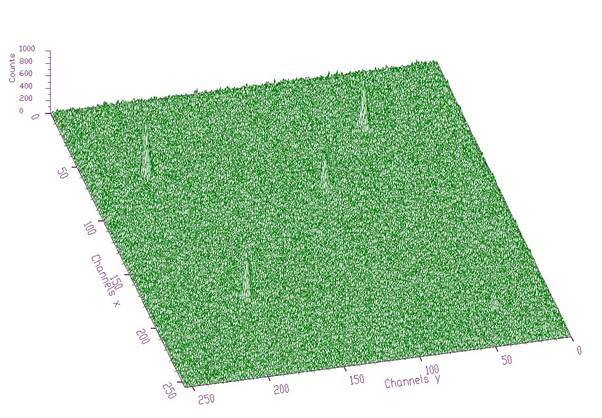Fig. 8 Peaks after subtraction of the estimated background (increasing clipping window with widths 8, 8 and algorithm based on one step filtering) from original two-dimensional gamma-gamma-ray spectrum (Fig. 6).  The artifacts from the above given Fig. 7 disappeared.

Script:

// Example to illustrate the background estimator (class TSpectrum).

// To execute this example, do

// root > .x Back_synt256.C

#include <TSpectrum>

void Back_synt256() {

Int_t i, j;

Double_t nbinsx = 64;

Double_t nbinsy = 64;

Double_t xmin  = 0;

Double_t xmax  = (Double_t)nbinsx;

Double_t ymin  = 0;

Double_t ymax  = (Double_t)nbinsy;

Float_t ** source = new float *[nbinsx];

for (i=0;i<nbinsx;i++)

source[i]=new float[nbinsy];

TH2F *back = new TH2F("back","Background estimation",nbinsx,xmin,xmax,nbinsy,ymin,ymax);

TFile *f = new TFile("spectra2\\TSpectrum2.root");

back=(TH2F*) f->Get("back3;1");

TCanvas *Background = new TCanvas("Background","Estimation of background with increasing window",10,10,1000,700);

TSpectrum *s = new TSpectrum();

for (i = 0; i < nbinsx; i++){

for (j = 0; j < nbinsy; j++){

source[i][j] = back->GetBinContent(i + 1,j + 1);

}

}

s->Background(source,nbinsx,nbinsy,8,8,kBackIncreasingWindow,kBackSuccessiveFiltering);//kBackOneStepFiltering

for (i = 0; i < nbinsx; i++){

for (j = 0; j < nbinsy; j++)

back->SetBinContent(i + 1,j + 1, source[i][j]);

}

back->Draw("SURF");

}

const char* SmoothMarkov(float** source, Int_t ssizex, Int_t ssizey, Int_t averWindow)
```   TWO-DIMENSIONAL MARKOV SPECTRUM SMOOTHING FUNCTION

This function calculates smoothed spectrum from source spectrum
based on Markov chain method.
The result is placed in the array pointed by source pointer.

Function parameters:
source-pointer to the array of source spectrum
ssizex-x length of source
ssizey-y length of source
averWindow-width of averaging smoothing window

```

Smoothing

Goal: Suppression of statistical fluctuations

         the algorithm is based on discrete Markov chain, which has very simple invariant distribution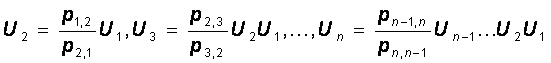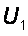being defined from the normalization condition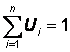n is the length of the smoothed spectrum and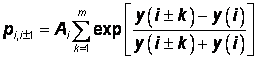is the probability of the change of the peak position from channel i to the channel i+1.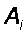is the normalization constant so that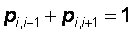and m is a width of smoothing window. We have extended this algortihm to two dimensions.

Function:

const char* TSpectrum2::SmoothMarkov(float **fSpectrum, int ssizex, int ssizey,  int averWindow)

This function calculates smoothed spectrum from the source spectrum based on Markov chain method. The result is placed in the vector pointed by source pointer. On successful completion it returns 0. On error it returns pointer to the string describing error.

Parameters:

fSpectrum-pointer to the matrix of source spectrum

ssizex, ssizey -lengths of the spectrum matrix

averWindow-width of averaging smoothing window

Reference:

 Z.K. Silagadze, A new algorithm for automatic photopeak searches. NIM A 376 (1996), 451.

```
```

Example 4  script Smooth.c :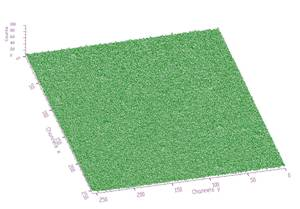Fig. 9 Original noisy spectrum.    Fig. 10 Smoothed spectrum m=3

Peaks can hardly be observed.     Peaks become apparent.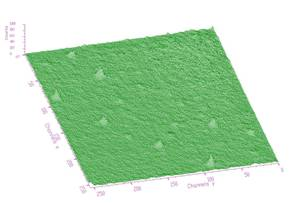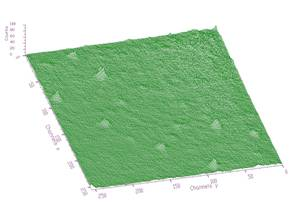Fig. 11 Smoothed spectrum m=5 Fig.12 Smoothed spectrum m=7

Script:

// Example to illustrate the Markov smoothing (class TSpectrum).

// To execute this example, do

// root > .x Smooth.C

#include <TSpectrum>

void Smooth() {

Int_t i, j;

Double_t nbinsx = 256;

Double_t nbinsy = 256;

Double_t xmin  = 0;

Double_t xmax  = (Double_t)nbinsx;

Double_t ymin  = 0;

Double_t ymax  = (Double_t)nbinsy;

Float_t ** source = new float *[nbinsx];

for (i=0;i<nbinsx;i++)

source[i]=new float[nbinsy];

TH2F *smooth = new TH2F("smooth","Background estimation",nbinsx,xmin,xmax,nbinsy,ymin,ymax);

TFile *f = new TFile("spectra2\\TSpectrum2.root");

smooth=(TH2F*) f->Get("smooth1;1");

TCanvas *Smoothing = new TCanvas("Smoothing","Markov smoothing",10,10,1000,700);

TSpectrum *s = new TSpectrum();

for (i = 0; i < nbinsx; i++){

for (j = 0; j < nbinsy; j++){

source[i][j] = smooth->GetBinContent(i + 1,j + 1);

}

}

s->SmoothMarkov(source,nbinsx,nbinsx,3);//5,7

for (i = 0; i < nbinsx; i++){

for (j = 0; j < nbinsy; j++)

smooth->SetBinContent(i + 1,j + 1, source[i][j]);

}

smooth->Draw("SURF");

}

const char * Deconvolution(float** source, float** resp, Int_t ssizex, Int_t ssizey, Int_t numberIterations, Int_t numberRepetitions, Double_t boost)
```   TWO-DIMENSIONAL DECONVOLUTION FUNCTION
This function calculates deconvolution from source spectrum
according to response spectrum
The result is placed in the matrix pointed by source pointer.

Function parameters:
source-pointer to the matrix of source spectrum
resp-pointer to the matrix of response spectrum
ssizex-x length of source and response spectra
ssizey-y length of source and response spectra
numberIterations, for details we refer to manual
numberRepetitions, for details we refer to manual
boost, boosting factor, for details we refer to manual

```

Deconvolution

Goal: Improvement of the resolution in spectra, decomposition of multiplets

Mathematical formulation of the 2-dimensional convolution system is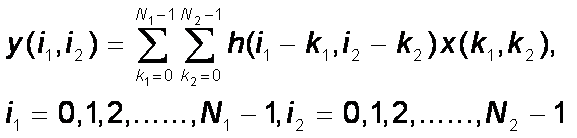where h(i,j) is the impulse response function, x, y are input and output matrices, respectively,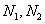are the lengths of x and h matrices

         let us assume that we know the response and the output matrices (spectra) of the above given system.

         the deconvolution represents solution of the overdetermined system of linear equations, i.e.,  the calculation of the matrix x.

         from numerical stability point of view the operation of deconvolution is extremely critical (ill-posed  problem) as well as time consuming operation.

         the Gold deconvolution algorithm proves to work very well even for 2-dimensional systems. Generalization of the algorithm for 2-dimensional systems was presented in , .

         for Gold deconvolution algorithm as well as for boosted deconvolution algorithm we refer also to TSpectrum

Function:

const char* TSpectrum2::Deconvolution(float **source, const float **resp, int ssizex, int ssizey, int numberIterations, int numberRepetitions, double boost)

This function calculates deconvolution from source spectrum according to response spectrum using Gold deconvolution algorithm. The result is placed in the matrix pointed by source pointer. On successful completion it returns 0. On error it returns pointer to the string describing error. If desired after every numberIterations one can apply boosting operation (exponential function with exponent given by boost coefficient) and repeat it numberRepetitions times.

Parameters:

source-pointer to the matrix of source spectrum

resp-pointer to the matrix of response spectrum

ssizex, ssizey-lengths of the spectrum matrix

numberIterations-number of iterations

numberRepetitions-number of repetitions for boosted deconvolution. It must be

greater or equal to one.

boost-boosting coefficient, applies only if numberRepetitions is greater than one.

Recommended range <1,2>.

References:

 M. Morháč, J. Kliman, V. Matouek, M. Veselský, I. Turzo.: Efficient one- and two-dimensional Gold deconvolution and its application to gamma-ray spectra decomposition. NIM, A401 (1997) 385-408.

 Morháč M., Matouek V., Kliman J., Efficient algorithm of multidimensional deconvolution and its application to nuclear data processing, Digital Signal Processing 13 (2003) 144.

```
```

Example 5  script Decon.c :

         response function (usually peak) should be shifted to the beginning of the coordinate system (see Fig. 13)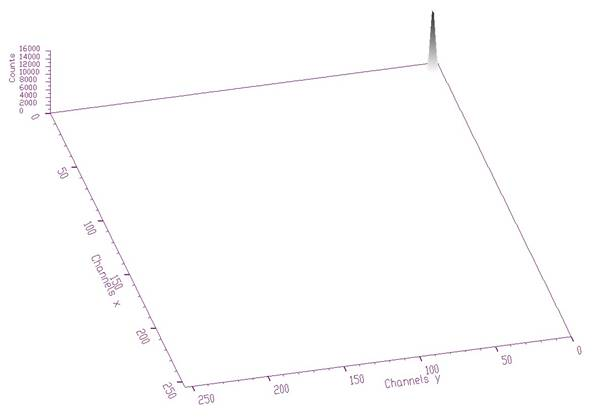Fig. 13 2-dimensional response spectrum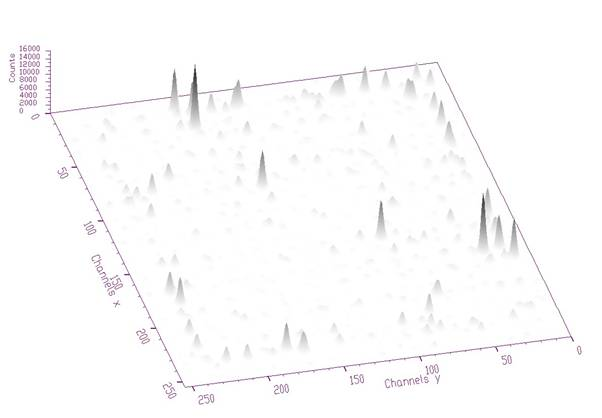Fig. 14 2-dimensional gamma-gamma-ray input spectrum (before deconvolution)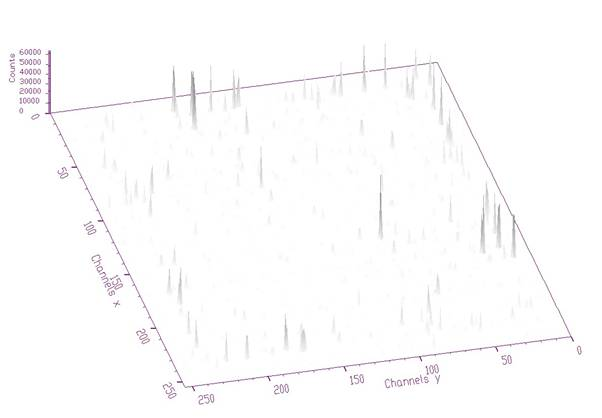Fig. 15 Spectrum from Fig. 14 after deconvolution (1000 iterations)

Script:

// Example to illustrate the Gold deconvolution (class TSpectrum2).

// To execute this example, do

// root > .x Decon.C

#include <TSpectrum2>

void Decon() {

Int_t i, j;

Double_t nbinsx = 256;

Double_t nbinsy = 256;

Double_t xmin  = 0;

Double_t xmax  = (Double_t)nbinsx;

Double_t ymin  = 0;

Double_t ymax  = (Double_t)nbinsy;

Float_t ** source = new float *[nbinsx];

for (i=0;i<nbinsx;i++)

source[i]=new float[nbinsy];

TH2F *decon = new TH2F("decon","Gold deconvolution",nbinsx,xmin,xmax,nbinsy,ymin,ymax);

TFile *f = new TFile("spectra2\\TSpectrum2.root");

decon=(TH2F*) f->Get("decon1;1");

Float_t ** response = new float *[nbinsx];

for (i=0;i<nbinsx;i++)

response[i]=new float[nbinsy];

TH2F *resp = new TH2F("resp","Response matrix",nbinsx,xmin,xmax,nbinsy,ymin,ymax);

resp=(TH2F*) f->Get("resp1;1");

TCanvas *Deconvol = new TCanvas("Deconvolution","Gold deconvolution",10,10,1000,700);

TSpectrum *s = new TSpectrum();

for (i = 0; i < nbinsx; i++){

for (j = 0; j < nbinsy; j++){

source[i][j] = decon->GetBinContent(i + 1,j + 1);

}

}

for (i = 0; i < nbinsx; i++){

for (j = 0; j < nbinsy; j++){

response[i][j] = resp->GetBinContent(i + 1,j + 1);

}

}

s->Deconvolution(source,response,nbinsx,nbinsy,1000,1,1);

for (i = 0; i < nbinsx; i++){

for (j = 0; j < nbinsy; j++)

decon->SetBinContent(i + 1,j + 1, source[i][j]);

}

decon->Draw("SURF");

}

Example 6  script Decon2.c :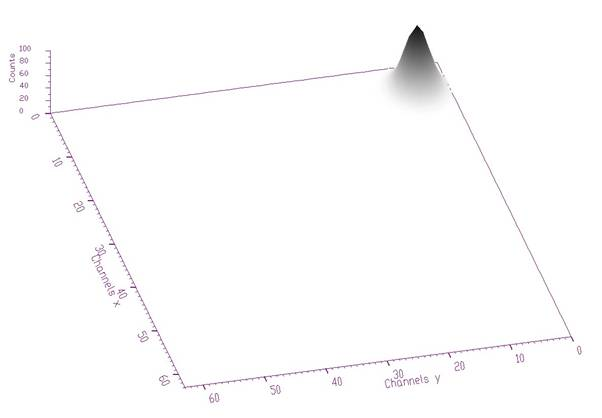Fig. 16 Response spectrum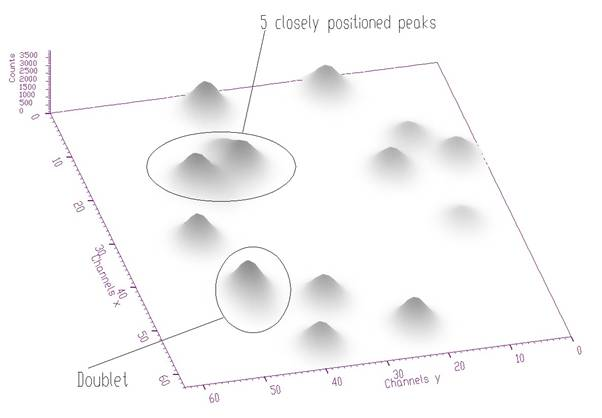Fig. 17 Original synthetic input spectrum (before deconvolution). It is composed of 17 peaks. 5 peaks are overlapping in the outlined multiplet and two peaks in doublet.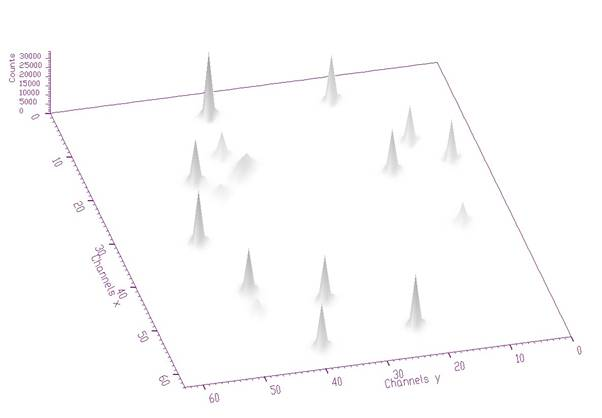Fig. 18 Spectrum from Fig. 17 after deconvolution (1000 iterations). Resolution is improved but the peaks in multiplet remained unresolved.

Script:

// Example to illustrate the Gold deconvolution (class TSpectrum2).

// To execute this example, do

// root > .x Decon2.C

#include <TSpectrum2>

void Decon2() {

Int_t i, j;

Double_t nbinsx = 64;

Double_t nbinsy = 64;

Double_t xmin  = 0;

Double_t xmax  = (Double_t)nbinsx;

Double_t ymin  = 0;

Double_t ymax  = (Double_t)nbinsy;

Float_t ** source = new float *[nbinsx];

for (i=0;i<nbinsx;i++)

source[i]=new float[nbinsy];

TH2F *decon = new TH2F("decon","Gold deconvolution",nbinsx,xmin,xmax,nbinsy,ymin,ymax);

TFile *f = new TFile("spectra2\\TSpectrum2.root");

decon=(TH2F*) f->Get("decon2;1");

Float_t ** response = new float *[nbinsx];

for (i=0;i<nbinsx;i++)

response[i]=new float[nbinsy];

TH2F *resp = new TH2F("resp","Response matrix",nbinsx,xmin,xmax,nbinsy,ymin,ymax);

resp=(TH2F*) f->Get("resp2;1");

TCanvas *Deconvol = new TCanvas("Deconvolution","Gold deconvolution",10,10,1000,700);

TSpectrum *s = new TSpectrum();

for (i = 0; i < nbinsx; i++){

for (j = 0; j < nbinsy; j++){

source[i][j] = decon->GetBinContent(i + 1,j + 1);

}

}

for (i = 0; i < nbinsx; i++){

for (j = 0; j < nbinsy; j++){

response[i][j] = resp->GetBinContent(i + 1,j + 1);

}

}

s->Deconvolution(source,response,nbinsx,nbinsy,1000,1,1);

for (i = 0; i < nbinsx; i++){

for (j = 0; j < nbinsy; j++)

decon->SetBinContent(i + 1,j + 1, source[i][j]);

}

decon->Draw("SURF");

}

Example 7  script Decon2HR.c :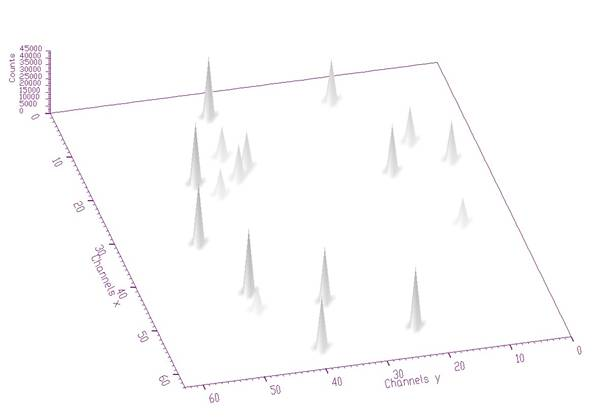Fig. 19 Spectrum from Fig. 17 after boosted deconvolution (50 iterations repeated 20 times, boosting coefficient was 1.2). All the peaks in multiplet as well as in doublet are completely decomposed.

Script:

// Example to illustrate boosted Gold deconvolution (class TSpectrum2).

// To execute this example, do

// root > .x Decon2HR.C

//#include <TSpectrum2>

void Decon2HR() {

Int_t i, j;

Double_t nbinsx = 64;

Double_t nbinsy = 64;

Double_t xmin  = 0;

Double_t xmax  = (Double_t)nbinsx;

Double_t ymin  = 0;

Double_t ymax  = (Double_t)nbinsy;

Float_t ** source = new float *[nbinsx];

for (i=0;i<nbinsx;i++)

source[i]=new float[nbinsy];

TH2F *decon = new TH2F("decon","Boosted Gold deconvolution",nbinsx,xmin,xmax,nbinsy,ymin,ymax);

TFile *f = new TFile("spectra2\\TSpectrum2.root");

decon=(TH2F*) f->Get("decon2;1");

Float_t ** response = new float *[nbinsx];

for (i=0;i<nbinsx;i++)

response[i]=new float[nbinsy];

TH2F *resp = new TH2F("resp","Response matrix",nbinsx,xmin,xmax,nbinsy,ymin,ymax);

resp=(TH2F*) f->Get("resp2;1");

TCanvas *Deconvol = new TCanvas("Deconvolution","Gold deconvolution",10,10,1000,700);

TSpectrum *s = new TSpectrum();

for (i = 0; i < nbinsx; i++){

for (j = 0; j < nbinsy; j++){

source[i][j] = decon->GetBinContent(i + 1,j + 1);

}

}

for (i = 0; i < nbinsx; i++){

for (j = 0; j < nbinsy; j++){

response[i][j] = resp->GetBinContent(i + 1,j + 1);

}

}

s->Deconvolution(source,response,nbinsx,nbinsy,1000,1,1);

for (i = 0; i < nbinsx; i++){

for (j = 0; j < nbinsy; j++)

decon->SetBinContent(i + 1,j + 1, source[i][j]);

}

decon->Draw("SURF");

}

Int_t SearchHighRes(float** source, float** dest, Int_t ssizex, Int_t ssizey, Double_t sigma, Double_t threshold, Bool_t backgroundRemove, Int_t deconIterations, Bool_t markov, Int_t averWindow)
```TWO-DIMENSIONAL HIGH-RESOLUTION PEAK SEARCH FUNCTION
This function searches for peaks in source spectrum
It is based on deconvolution method. First the background is
removed (if desired), then Markov spectrum is calculated
(if desired), then the response function is generated
according to given sigma and deconvolution is carried out.

Function parameters:
source-pointer to the matrix of source spectrum
dest-pointer to the matrix of resulting deconvolved spectrum
ssizex-x length of source spectrum
ssizey-y length of source spectrum
sigma-sigma of searched peaks, for details we refer to manual
threshold-threshold value in % for selected peaks, peaks with
amplitude less than threshold*highest_peak/100
are ignored, see manual
backgroundRemove-logical variable, set if the removal of
background before deconvolution is desired
deconIterations-number of iterations in deconvolution operation
markov-logical variable, if it is true, first the source spectrum
is replaced by new spectrum calculated using Markov
chains method.
averWindow-averanging window of searched peaks, for details
we refer to manual (applies only for Markov method)

```

Peaks searching

Goal: to identify automatically the peaks in spectrum with the presence of the continuous background, one-fold coincidences (ridges) and statistical fluctuations - noise.

The common problems connected with correct peak identification in two-dimensional coincidence spectra are

• non-sensitivity to noise, i.e., only statistically relevant peaks should be identified
• non-sensitivity of the algorithm to continuous background
• non-sensitivity to one-fold coincidences (coincidences peak  background in both dimensions) and their crossings
• ability to identify peaks close to the edges of the spectrum region. Usually peak finders fail to detect them
• resolution, decomposition of doublets and multiplets. The algorithm should be able to recognize close positioned peaks.
• ability to identify peaks with different sigma

Function:

TSpectrum2::SearchHighRes (float **source,float **dest, int ssizex, int ssizey, float sigma, double threshold, bool backgroundRemove,int deconIterations, bool markov, int averWindow)

This function searches for peaks in source spectrum. It is based on deconvolution method. First the background is removed (if desired), then Markov smoothed spectrum is calculated (if desired), then the response function is generated according to given sigma and deconvolution is carried out. The order of peaks is arranged according to their heights in the spectrum after background elimination. The highest peak is the first in the list. On success it returns number of found peaks.

Parameters:

source-pointer to the matrix of source spectrum

dest-resulting spectrum after deconvolution

ssizex, ssizey-lengths of the source and destination spectra

sigma-sigma of searched peaks

threshold- threshold value in % for selected peaks, peaks with amplitude less than threshold*highest_peak/100 are ignored

backgroundRemove- background_remove-logical variable, true if the removal of background before deconvolution is desired

deconIterations-number of iterations in deconvolution operation

markov-logical variable, if it is true, first the source spectrum is replaced by new spectrum calculated using Markov chains method

averWindow-width of averaging smoothing window

References:

 M.A. Mariscotti: A method for identification of peaks in the presence of background and its application to spectrum analysis. NIM 50 (1967), 309-320.

  M. Morháč, J. Kliman, V. Matouek, M. Veselský, I. Turzo.:Identification of peaks in multidimensional coincidence gamma-ray spectra. NIM, A443 (2000) 108-125.

 Z.K. Silagadze, A new algorithm for automatic photopeak searches. NIM A 376 (1996), 451.

```
```

Examples of peak searching method

SearchHighRes function provides users with the possibility to vary the input parameters and with the access to the output deconvolved data in the destination spectrum. Based on the output data one can tune the parameters.

Example 8  script Src.c: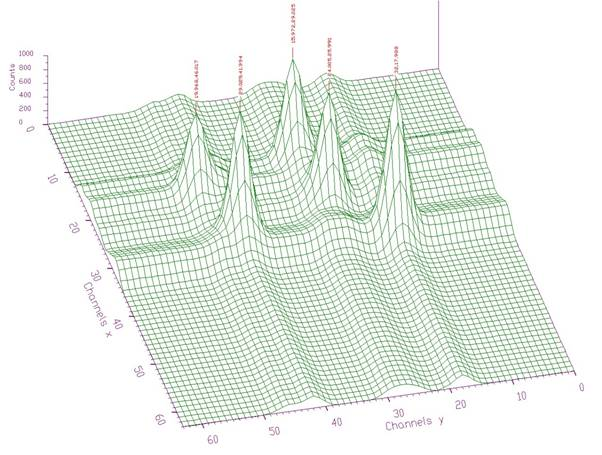Fig. 20 Two-dimensional spectrum with found peaks denoted by markers (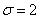, threshold=5%, 3 iterations steps in the deconvolution)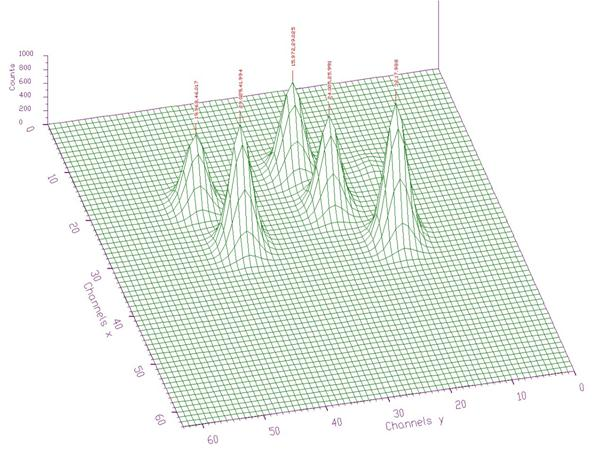Fig. 21 Spectrum from Fig. 20 after background elimination and deconvolution

Script:

// Example to illustrate high resolution peak searching function (class TSpectrum).

// To execute this example, do

// root > .x Src.C

#include <TSpectrum2>

void Src() {

Int_t i, j, nfound;

Double_t nbinsx = 64;

Double_t nbinsy = 64;

Double_t xmin  = 0;

Double_t xmax  = (Double_t)nbinsx;

Double_t ymin  = 0;

Double_t ymax  = (Double_t)nbinsy;

Float_t ** source = new float *[nbinsx];

for (i=0;i<nbinsx;i++)

source[i]=new float[nbinsy];

Float_t ** dest = new float *[nbinsx];

for (i=0;i<nbinsx;i++)

dest[i]=new float[nbinsy];

TH2F *search = new TH2F("search","High resolution peak searching",nbinsx,xmin,xmax,nbinsy,ymin,ymax);

TFile *f = new TFile("spectra2\\TSpectrum2.root");

search=(TH2F*) f->Get("search4;1");

TCanvas *Searching = new TCanvas("Searching","High resolution peak searching",10,10,1000,700);

TSpectrum2 *s = new TSpectrum2();

for (i = 0; i < nbinsx; i++){

for (j = 0; j < nbinsy; j++){

source[i][j] = search->GetBinContent(i + 1,j + 1);

}

}

nfound = s->SearchHighRes(source, dest, nbinsx, nbinsy, 2, 5, kTRUE, 3, kFALSE, 3);

printf("Found %d candidate peaks\n",nfound);

for(i=0;i<nfound;i++)

printf("posx= %d, posy= %d, value= %d\n",(int)(fPositionX[i]+0.5), (int)(fPositionY[i]+0.5), (int)source[(int)(fPositionX[i]+0.5)][(int)(fPositionY[i]+0.5)]);

}

Example 9  script Src2.c: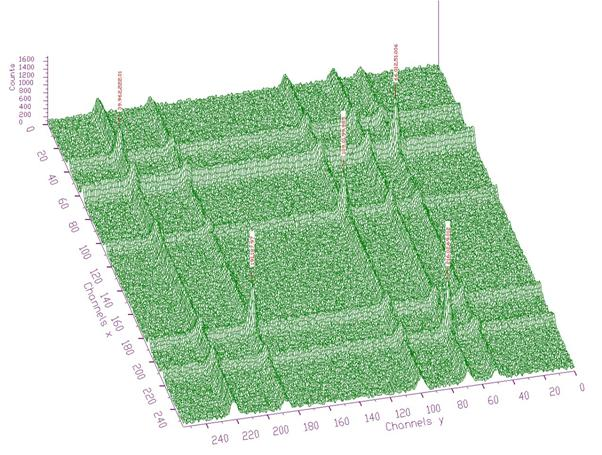Fig. 22 Two-dimensional noisy spectrum with found peaks denoted by markers (, threshold=10%, 10 iterations steps in the deconvolution). One can observe that the algorithm is insensitive to the crossings of one-dimensional ridges. It identifies only two-cooincidence peaks.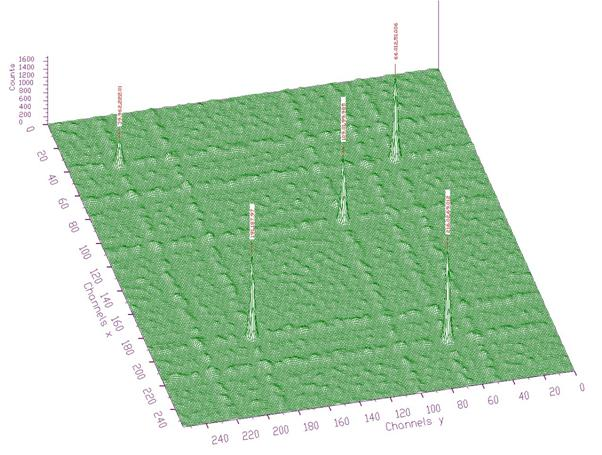Fig. 23 Spectrum from Fig. 22 after background elimination and deconvolution

Script:

// Example to illustrate high resolution peak searching function (class TSpectrum).

// To execute this example, do

// root > .x Src2.C

#include <TSpectrum2>

void Src2() {

Int_t i, j, nfound;

Double_t nbinsx = 256;

Double_t nbinsy = 256;

Double_t xmin  = 0;

Double_t xmax  = (Double_t)nbinsx;

Double_t ymin  = 0;

Double_t ymax  = (Double_t)nbinsy;

Float_t ** source = new float *[nbinsx];

for (i=0;i<nbinsx;i++)

source[i]=new float[nbinsy];

Float_t ** dest = new float *[nbinsx];

for (i=0;i<nbinsx;i++)

dest[i]=new float[nbinsy];

TH2F *search = new TH2F("search","High resolution peak searching",nbinsx,xmin,xmax,nbinsy,ymin,ymax);

TFile *f = new TFile("spectra2\\TSpectrum2.root");

search=(TH2F*) f->Get("back3;1");

TCanvas *Searching = new TCanvas("Searching","High resolution peak searching",10,10,1000,700);

TSpectrum2 *s = new TSpectrum2();

for (i = 0; i < nbinsx; i++){

for (j = 0; j < nbinsy; j++){

source[i][j] = search->GetBinContent(i + 1,j + 1);

}

}

nfound = s->SearchHighRes(source, dest, nbinsx, nbinsy, 2, 10, kTRUE, 10, kFALSE, 3);

printf("Found %d candidate peaks\n",nfound);

for(i=0;i<nfound;i++)

printf("posx= %d, posy= %d, value= %d\n",(int)(fPositionX[i]+0.5), (int)(fPositionY[i]+0.5), (int)source[(int)(fPositionX[i]+0.5)][(int)(fPositionY[i]+0.5)]);

}

Example 10  script Src3.c: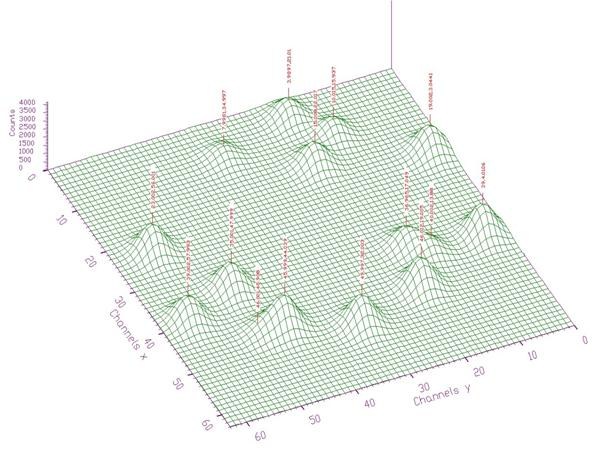Fig. 24 Two-dimensional spectrum with 15 found peaks denoted by markers. Some peaks are positioned close to each other. It is necessary to increase number of iterations in the deconvolution. In next 3 Figs. we shall study the influence of this parameter.Fig. 25 Spectrum from Fig. 24 after deconvolution (# of iterations = 3). Number of identified peaks = 13.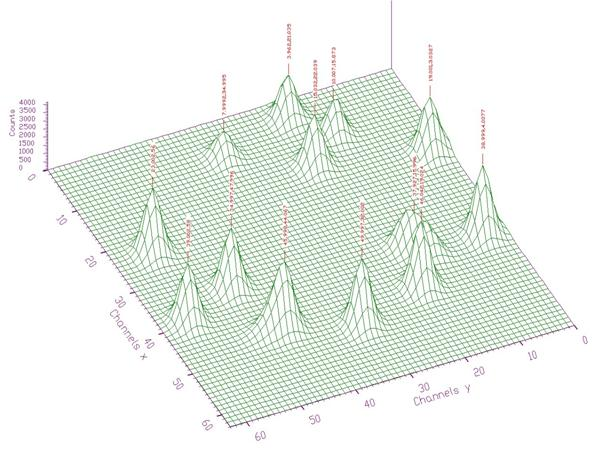Fig. 26 Spectrum from Fig. 24 after deconvolution (# of iterations = 10). Number of identified peaks = 13.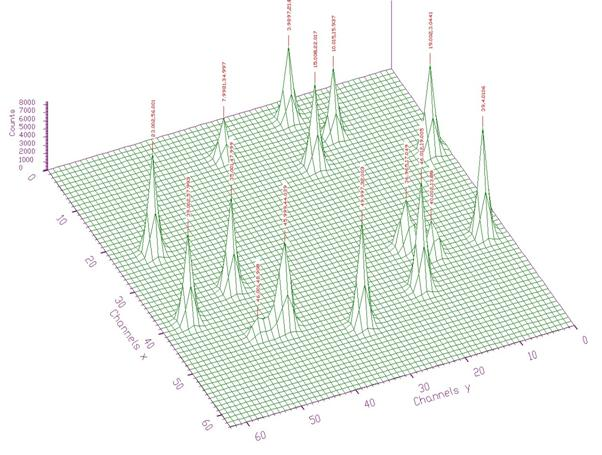Fig. 27 Spectrum from Fig. 24 after deconvolution (# of iterations = 100). Number of identified peaks = 15. Now the algorithm is able to decompose two doublets in the spectrum.

Script:

// Example to illustrate high resolution peak searching function (class TSpectrum).

// To execute this example, do

// root > .x Src3.C

#include <TSpectrum2>

void Src3() {

Int_t i, j, nfound;

Double_t nbinsx = 64;

Double_t nbinsy = 64;

Double_t xmin  = 0;

Double_t xmax  = (Double_t)nbinsx;

Double_t ymin  = 0;

Double_t ymax  = (Double_t)nbinsy;

Float_t ** source = new float *[nbinsx];

for (i=0;i<nbinsx;i++)

source[i]=new float[nbinsy];

Float_t ** dest = new float *[nbinsx];

for (i=0;i<nbinsx;i++)

dest[i]=new float[nbinsy];

TH2F *search = new TH2F("search","High resolution peak searching",nbinsx,xmin,xmax,nbinsy,ymin,ymax);

TFile *f = new TFile("spectra2\\TSpectrum2.root");

search=(TH2F*) f->Get("search1;1");

TCanvas *Searching = new TCanvas("Searching","High resolution peak searching",10,10,1000,700);

TSpectrum2 *s = new TSpectrum2();

for (i = 0; i < nbinsx; i++){

for (j = 0; j < nbinsy; j++){

source[i][j] = search->GetBinContent(i + 1,j + 1);

}

}

nfound = s->SearchHighRes(source, dest, nbinsx, nbinsy, 2, 2, kFALSE, 3, kFALSE, 1);//3, 10, 100

printf("Found %d candidate peaks\n",nfound);

for(i=0;i<nfound;i++)

printf("posx= %d, posy= %d, value= %d\n",(int)(fPositionX[i]+0.5), (int)(fPositionY[i]+0.5), (int)source[(int)(fPositionX[i]+0.5)][(int)(fPositionY[i]+0.5)]);

}

Example 11  script Src4.c: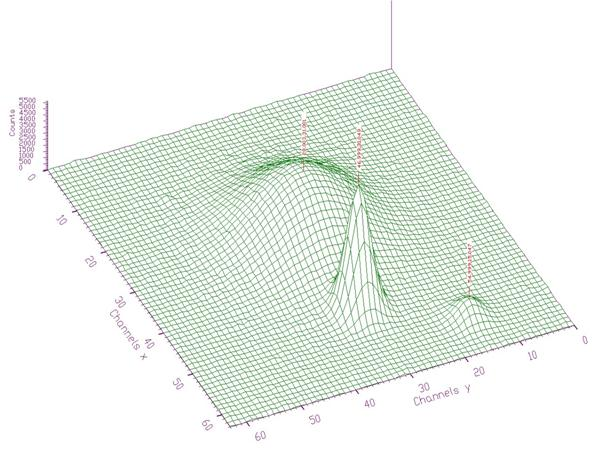Fig. 28 Two-dimensional spectrum with peaks with different sigma denoted by markers (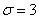, threshold=5%, 10 iterations steps in the deconvolution, Markov smoothing with window=3)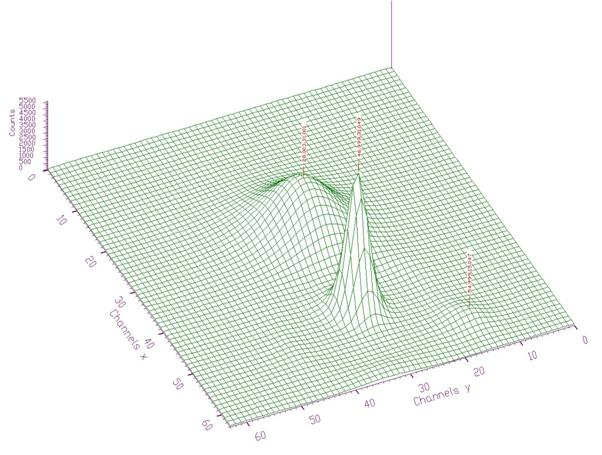Fig. 29 Spectrum from Fig. 28 after smoothing and deconvolution.

Script:

// Example to illustrate high resolution peak searching function (class TSpectrum).

// To execute this example, do

// root > .x Src4.C

#include <TSpectrum2>

void Src4() {

Int_t i, j, nfound;

Double_t nbinsx = 64;

Double_t nbinsy = 64;

Double_t xmin  = 0;

Double_t xmax  = (Double_t)nbinsx;

Double_t ymin  = 0;

Double_t ymax  = (Double_t)nbinsy;

Float_t ** source = new float *[nbinsx];

for (i=0;i<nbinsx;i++)

source[i]=new float[nbinsy];

Float_t ** dest = new float *[nbinsx];

for (i=0;i<nbinsx;i++)

dest[i]=new float[nbinsy];

TH2F *search = new TH2F("search","High resolution peak searching",nbinsx,xmin,xmax,nbinsy,ymin,ymax);

TFile *f = new TFile("spectra2\\TSpectrum2.root");

search=(TH2F*) f->Get("search2;1");

TCanvas *Searching = new TCanvas("Searching","High resolution peak searching",10,10,1000,700);

TSpectrum2 *s = new TSpectrum2();

for (i = 0; i < nbinsx; i++){

for (j = 0; j < nbinsy; j++){

source[i][j] = search->GetBinContent(i + 1,j + 1);

}

}

nfound = s->SearchHighRes(source, dest, nbinsx, nbinsy, 3, 5, kFALSE, 10, kTRUE, 3);

printf("Found %d candidate peaks\n",nfound);

for(i=0;i<nfound;i++)

printf("posx= %d, posy= %d, value= %d\n",(int)(fPositionX[i]+0.5), (int)(fPositionY[i]+0.5), (int)source[(int)(fPositionX[i]+0.5)][(int)(fPositionY[i]+0.5)]);

}

Example 12  script Src5.c: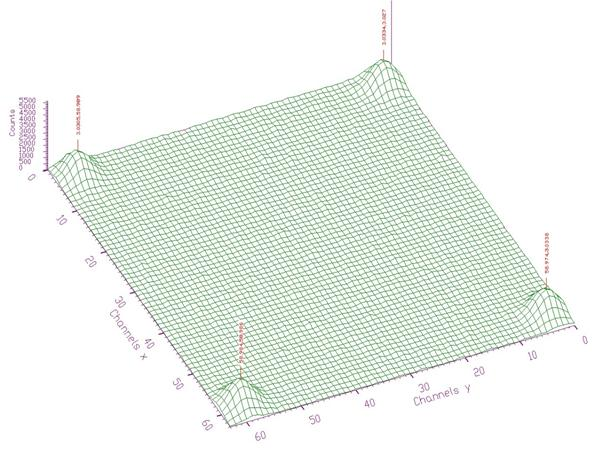Fig. 30 Two-dimensional spectrum with peaks positioned close to the edges denoted by markers (, threshold=5%, 10 iterations steps in the deconvolution)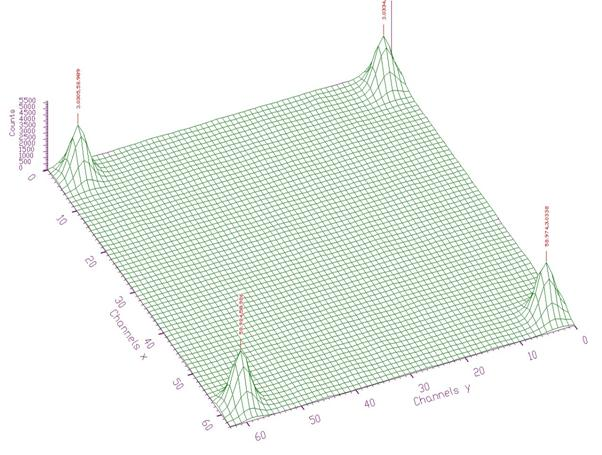Fig. 31 Spectrum from Fig. 30 after deconvolution.

Script:

// Example to illustrate high resolution peak searching function (class TSpectrum).

// To execute this example, do

// root > .x Src5.C

#include <TSpectrum2>

void Src5() {

Int_t i, j, nfound;

Double_t nbinsx = 64;

Double_t nbinsy = 64;

Double_t xmin  = 0;

Double_t xmax  = (Double_t)nbinsx;

Double_t ymin  = 0;

Double_t ymax  = (Double_t)nbinsy;

Float_t ** source = new float *[nbinsx];

for (i=0;i<nbinsx;i++)

source[i]=new float[nbinsy];

Float_t ** dest = new float *[nbinsx];

for (i=0;i<nbinsx;i++)

dest[i]=new float[nbinsy];

TH2F *search = new TH2F("search","High resolution peak searching",nbinsx,xmin,xmax,nbinsy,ymin,ymax);

TFile *f = new TFile("spectra2\\TSpectrum2.root");

search=(TH2F*) f->Get("search3;1");

TCanvas *Searching = new TCanvas("Searching","High resolution peak searching",10,10,1000,700);

TSpectrum2 *s = new TSpectrum2();

for (i = 0; i < nbinsx; i++){

for (j = 0; j < nbinsy; j++){

source[i][j] = search->GetBinContent(i + 1,j + 1);

}

}

nfound = s->SearchHighRes(source, dest, nbinsx, nbinsy, 2, 5, kFALSE, 10, kFALSE, 1);

printf("Found %d candidate peaks\n",nfound);

for(i=0;i<nfound;i++)

printf("posx= %d, posy= %d, value= %d\n",(int)(fPositionX[i]+0.5), (int)(fPositionY[i]+0.5), (int)source[(int)(fPositionX[i]+0.5)][(int)(fPositionY[i]+0.5)]);

}

Int_t StaticSearch(const TH1* hist, Double_t sigma = 2, Option_t* option = "goff", Double_t threshold = 0.05)
```static function, interface to TSpectrum2::Search
```
TH1 * StaticBackground(const TH1* hist, Int_t niter = 20, Option_t* option = "")
```static function, interface to TSpectrum2::Background
```
TH1 * GetHistogram() const
`{return fHistogram;}`
Int_t GetNPeaks() const
`{return fNPeaks;}`
Float_t * GetPositionX() const
`{return fPositionX;}`
Float_t * GetPositionY() const
`{return fPositionY;}`